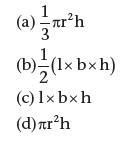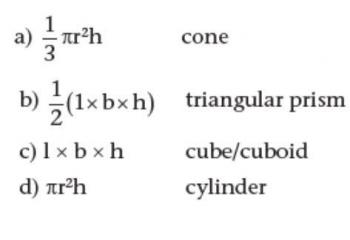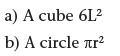# Chapter 6 – Measures

Consider the following statements and click to reveal the answer.

1. The weight of an average man is 72 kg. What will it be on the moon?

12kg

2. The volume of a box is 400 cubic centimetres. What is its capacity?

400 ml

3. What are the three important stages of development in children’s under­standing of measurement identified by the National Curriculum?

The three important stages of development in children’s understanding of measurement are:

• direct comparison using matching, with no actual measuring;
• using non-standard units;
• using standard units.

4. Name the two different systems of units of measurement used in the UK.

Metric and Imperial.

5. The SI determines the units of measurement in the metric system. What does SI stand for?

System International

6. Which aspect of measurement uses the same units in both systems?

The aspect of measurement that uses the same units in both systems is Time – seconds, minutes, hours.

7. Complete the following table to show the units of measurement: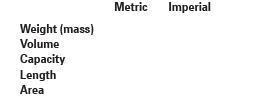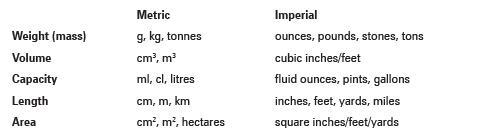8. Complete the following approximate equivalences: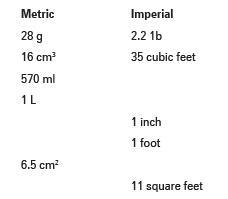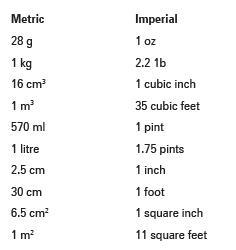9. The following formulae give the volumes of which regular 3-D shapes?